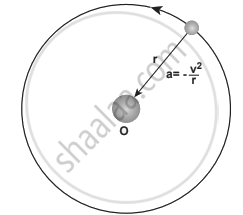Tamil Nadu Board of Secondary EducationHSC Science Class 11th

# Explain how Newton arrived at his law of gravitation from Kepler’s third law. - Physics

Explain how Newton arrived at his law of gravitation from Kepler’s third law.

#### Solution

Newton considered the orbits of the planets as circular. For a circular orbit of radius r, the centripetal acceleration towards the center is

a = -"v"^2/"r" ........(1)Point mass orbiting in a circular orbit

Here v is the velocity and r, the distance of the planet from the center of the orbit.

The velocity in terms of known quantities r and T is

v = (2π"r")/"T" .......(2)

Here T is the time period of the revolution of the planet. Substituting this value of ‘v’ in equation (1) we get,

a = -((2π"r")/"T")^2/"r"

= -(4π^2"r")/"T"^2 ........(3)

Substituting the value of ‘a’ from (3) in Newton’s second law, F = ma where ‘m’ is the mass of the planet.

F = -(4π^2"mr")/"T"^2 .......(4)

From Kepler’s third law,

"r"^3/"T"^2 = k (constant) ......(5)

"r"/"T"^2 = "k"/"r"^2 .....(6)

By using equation (6) in the force expression, we can arrive at the law of gravitation.

F = -(4π^2"mk")/"r"^2 .......(7)

Here negative sign implies that the force is attractive and it acts towards the center. He equated the constant 4π2k to GM which turned out to be the law of gravitation.

F = -"GMm"/"r"^2

Concept: Elementary Ideas of Astronomy
Is there an error in this question or solution?

Share